## How to convert week number to date or vice versa in Excel?

Is there a way to get the week number from a given date or extract the date range from a specific week number and year in Excel? To solve this task, the following formulas may do you a favor.

Convert week number to date with formulas

Convert date to week number with formulas

#### Convert week number to date with formulas

Supposing I have a random year and week number which are 2015 and 15 in a worksheet as following screenshot shown, and now I want to find out the specific dates from Monday to Sunday by this given week number.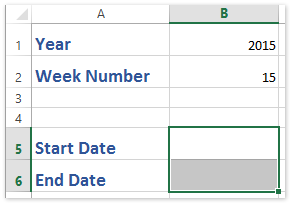To calculate the date range by the specific week number, you can use the following formulas:

1. Select a blank cell you will return the start date (in our case we select the Cell B5), enter the formula: =MAX(DATE(B1,1,1),DATE(B1,1,1)-WEEKDAY(DATE(B1,1,1),2)+(B2-1)*7+1), and press the Enter key. See screenshot below:

2. Select another blank cell you will return the end date (in our case we select the Cell B6), enter =MIN(DATE(B1+1,1,0),DATE(B1,1,1)-WEEKDAY(DATE(B1,1,1),2)+B2*7), and press the Enter key. See screenshot below: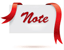Formula is too complicated to remember? Save the formula as an Auto Text entry for reusing with only one click in future! Read more…     Free trial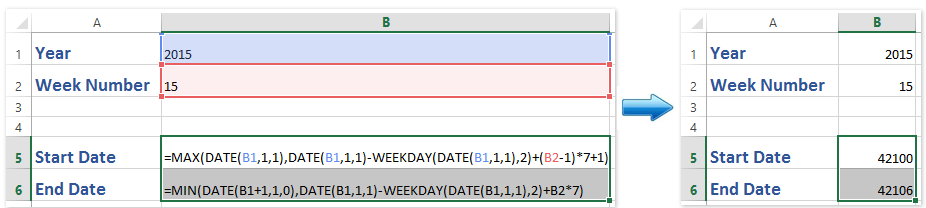Note: In both formulas above, B1 contains a year and B2 is a specified week number, you can change the arguments to your need).

3. As you see, both formulas return numbers instead of dates. Keep selecting both calculating results, and click Home > Number Format box > Short Date to change the numbers to dates. See screenshot below: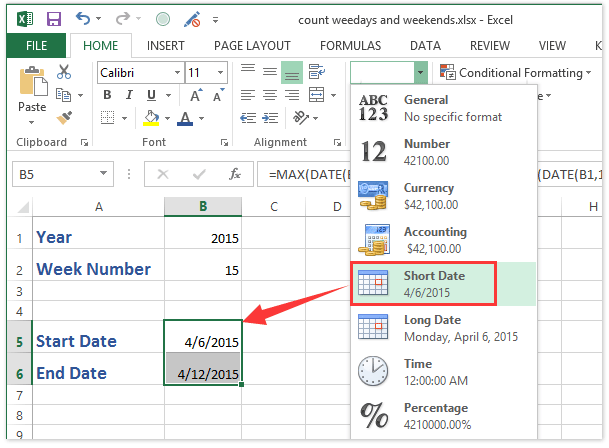#### One click to convert multiple non-standard formatting dates/numbers/text to normal dates in Excel

Kutools for Excel's Convert to Date utility can help you easily identify and convert non-standard dates or numbers (yyyymmdd) or text to normal dates with only one click in Excel.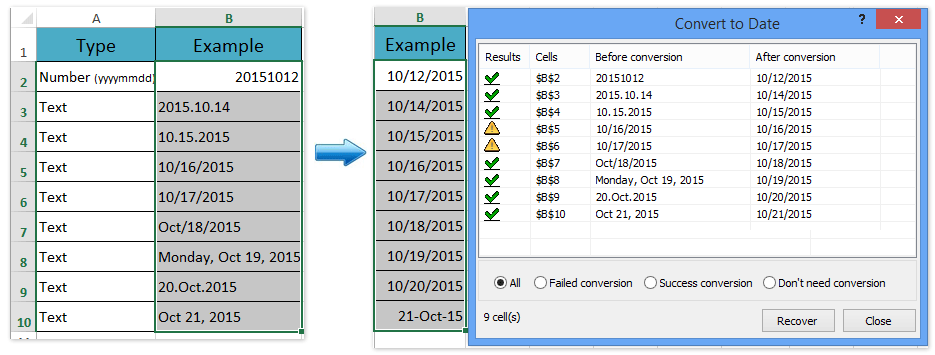#### Convert date to week number with formulas

On the other hand, you can also apply the WEEKNUM function to convert a date to corresponding week number.

1. Select a blank cell you will return the week number, enter this formula: =WEEKNUM(B1,1), and press the Enter key. See screenshot: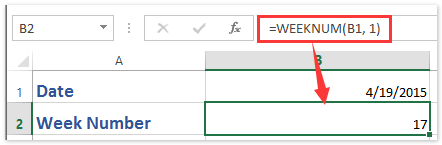Notes:

(1) In above formula, B1 contains the date that you want to use.

(2) If you need to return the week number from a date which begins in Monday, please apply this formula: =WEEKNUM(B1,2).

#### Related articles:

How to count the number of specific weekdays between two dates in Excel?

How to add / subtract days / months / years to date in Excel?

### Best Office Productivity Tools

 Popular Features: Find, Highlight or Identify Duplicates   |  Delete Blank Rows   |  Combine Columns or Cells without Losing Data   |   Round without Formula ... Super Lookup: Multiple Criteria VLookup  |   Multiple Value VLookup  |   VLookup Across Multiple Sheets   |   Fuzzy Lookup .... Advanced Drop-down List: Quickly Create Drop Down List   |  Dependent Drop Down List   |  Multi-select Drop Down List .... Column Manager: Add a Specific Number of Columns  |  Move Columns  |  Toggle Visibility Status of Hidden Columns  |  Compare Ranges & Columns ... Featured Features: Grid Focus   |  Design View   |   Big Formula Bar   |  Workbook & Sheet Manager   |  Resource Library (Auto Text)   |  Date Picker   |  Combine Worksheets   |  Encrypt/Decrypt Cells   |  Send Emails by List   |  Super Filter   |   Special Filter (filter bold/italic/strikethrough...) ... Top 15 Toolsets:  12 Text Tools (Add Text, Remove Characters, ...)   |   50+ Chart Types (Gantt Chart, ...)   |   40+ Practical Formulas (Calculate age based on birthday, ...)   |   19 Insertion Tools (Insert QR Code, Insert Picture from Path, ...)   |   12 Conversion Tools (Numbers to Words, Currency Conversion, ...)   |   7 Merge & Split Tools (Advanced Combine Rows, Split Cells, ...)   |   ... and more

Supercharge Your Excel Skills with Kutools for Excel, and Experience Efficiency Like Never Before. Kutools for Excel Offers Over 300 Advanced Features to Boost Productivity and Save Time.  Click Here to Get The Feature You Need The Most...#### Office Tab Brings Tabbed interface to Office, and Make Your Work Much Easier

• Enable tabbed editing and reading in Word, Excel, PowerPoint, Publisher, Access, Visio and Project.
• Open and create multiple documents in new tabs of the same window, rather than in new windows.
• Increases your productivity by 50%, and reduces hundreds of mouse clicks for you every day!No ratings yet. Be the first to rate!
This comment was minimized by the moderator on the site
All of these formulas have issues when the date is in the last week of the year. They don't give the correct date for Monday of the last week.
This comment was minimized by the moderator on the site
I find this better "Start of week"
B5=(8-WEEKDAY(DATE(B1,1,1),1))+((B2-2)*7)+DATE(B1,1,1)

8-WEEKDAY(DATE(B1,1,1),1) => find the no. of days in a week for first week of the year
(B2-2)*7 => calculate the number of days excluding the first week of year and the week for which calculation is being done
Then add these 2 to the first day of the year to get first day of the desired week

Then "End of week",
B6=B5+6

PS:
Week starts on Sunday
For weeks starting on Monday, use this instead:
B5=(8-WEEKDAY(DATE(B1,1,1),2))+((B2-2)*7)+DATE(B1,1,1)
This comment was minimized by the moderator on the site
Your formula works perfectly for every year. End of week would be B6=B5+6 though. Thanks a lot!
This comment was minimized by the moderator on the site
Thanks. Will correct that...
This comment was minimized by the moderator on the site
This formula is overly complicated. If you have a date say 8/17/2021 in Cell A1, to get the Week Ending(as of Saturday) you just need the following: = A1-WEEKDAY(A1,1)+7This will return 8/21/2021. Date of 12/30/2020 will return 1/2/2021 as week ending.
This comment was minimized by the moderator on the site
Hi,

not sure if this has been asked, but essentially, I want to be able to drag the date and the week number automatically fill beside it when I do that.

Can anyone help?

This comment was minimized by the moderator on the site
@gilly2801 you can use an array formula for example with "=weeknum(C2:C)" press command shift enter and it will turn it into an array function.
This comment was minimized by the moderator on the site

Suppose we are considering Date 1 to 7 is week 1 and 8 to 14 is week 2. Can you please help me out how can i use if function to calculate week. I have tried but not able to get the correct result.
This comment was minimized by the moderator on the site
=ROUNDUP((TODAY()-DATE(YEAR(TODAY()),1,1))/7,0)
This comment was minimized by the moderator on the site
=ROUNDUP((TODAY()-DATE(YEAR(TODAY()),1,1))/7,0)
This comment was minimized by the moderator on the site
Thank YOU!!!!
This comment was minimized by the moderator on the site
Thank you very much!

=CONCATENATE("Inclusive Dates: ",TEXT(MAX(DATE(TEXT(TODAY(),"yyyy"),1,1),DATE(TEXT(TODAY(),"yyyy"),1,1)-WEEKDAY(DATE(TEXT(TODAY(),"yyyy"),1,1),2)+(WEEKNUM(TODAY())-1)*7+1),"MMMM")," ",TEXT(MAX(DATE(TEXT(TODAY(),"yyyy"),1,1),DATE(TEXT(TODAY(),"yyyy"),1,1)-WEEKDAY(DATE(TEXT(TODAY(),"yyyy"),1,1),2)+(WEEKNUM(TODAY())-1)*7+1),"DD"),", ",TEXT(MAX(DATE(TEXT(TODAY(),"yyyy"),1,1),DATE(TEXT(TODAY(),"yyyy"),1,1)-WEEKDAY(DATE(TEXT(TODAY(),"yyyy"),1,1),2)+(WEEKNUM(TODAY())-1)*7+1),"YYYY")," - ",TEXT(MIN(DATE(TEXT(TODAY(),"yyyy")+1,1,0),DATE(TEXT(TODAY(),"yyyy"),1,1)-WEEKDAY(DATE(TEXT(TODAY(),"yyyy"),1,1),2)+WEEKNUM(TODAY())*7),"MMMM")," ",TEXT(MIN(DATE(TEXT(TODAY(),"yyyy")+1,1,0),DATE(TEXT(TODAY(),"yyyy"),1,1)-WEEKDAY(DATE(TEXT(TODAY(),"yyyy"),1,1),2)+WEEKNUM(TODAY())*7),"DD"),", ",TEXT(MIN(DATE(TEXT(TODAY(),"yyyy")+1,1,0),DATE(TEXT(TODAY(),"yyyy"),1,1)-WEEKDAY(DATE(TEXT(TODAY(),"yyyy"),1,1),2)+WEEKNUM(TODAY())*7),"YYYY"))
This comment was minimized by the moderator on the site
Date(2017;1;7 * weeknumer - 5) 5 monday, 4 tuesday... :)
This comment was minimized by the moderator on the site
Or to make in not specific to the year 2017...
=DATE(YEAR,1,7 * WEEKNUM - WEEKDAY(DATE(YEAR,1,7) - 2))

2 monday, 3 tuesday,...
This comment was minimized by the moderator on the site
I'm sorry folks, but I could neither understand nor make work any of the formulas above so I finally figured out the following solution: DATE(B1,1,1)+(\$A4×7)−(6−(7−WEEKDAY(DATE(B1,1,1),first-day))) The first part "DATE(B1,1,1)+(B2×7)" simply takes January 1 of the year and adds the number of weeks. The next part calculates how many days to subtract from the WEEKDAY of January 1 to get the first day of the week. This is what I finally figured out: 6−(7−WEEKDAY(DATE(B1,1,1),first-day)) If, for example, January 1 falls on a Sunday (day 7), then this formula become "6-(7-7)" or simply 6 - which is the number of days you need to subtract to get Monday of that week. Try other days. Finally, if you want to find any other day of the week, just add the WEEKDAY number minus 1 to this result. So the last day of the week (Sunday) is: DATE(B1,1,1)+(\$A4×7)−(6−(7−WEEKDAY(DATE(B1,1,1),first-day)))+(7−1) Which can be simplified to: DATE(B1,1,1)+(\$A4×7)−(12−(7−WEEKDAY(DATE(B1,1,1),first-day))) Wednesday would be: DATE(B1,1,1)+(\$A4×7)−(8−(7−WEEKDAY(DATE(B1,1,1),first-day))) etc. I hope this helps someone else who needs this both with a workable solution along with a bit of understanding of how it was arrived at!
This comment was minimized by the moderator on the site
It's a great formulas and ideal! However, it's important to point out that Excel default system week number counts from Sunday to Saturday. Adjustment is needed if the week number is extracted from Excel Weeknumber formulas.
There are no comments posted here yet